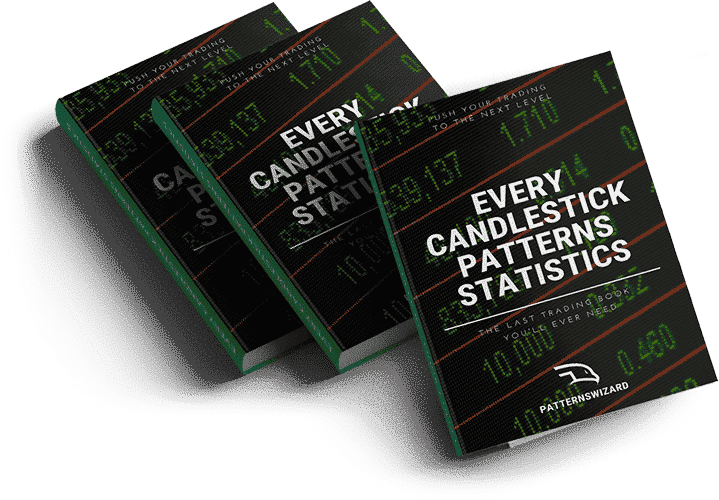# Quick Guide to Mastering the Risk/Reward Ratio• The Risk/Reward ratio is a measure of the potential profit potential of trade compared to its risk.
• It a very important metric used by traders to manage their risk on each trade they consider.
• With win rate it helps estimate how profitable a strategy is.

There are many components that are parts of successful trading and are parts of the DNA of a successful trader. Understanding of all these components is imperative in order to succeed in trading. To put it in simple words, it is the prerequisite of trading in a constantly changing and high paced financial markets. Among these components, risk/reward (R/R) ration is extremely important. Understanding of the risk/reward ratio is actually a key to success.

The risk/reward ratio (also called R/R ratio) defines the perspective reward for an investor for every dollar he/she risks and invests in a financial market. The R/R ratio also helps investors to compare the expected return of an investment and the maximum risk they must take to achieve these returns. Traders also use risk/reward ratio to calculate capital and risk of loss during trading. Therefore, it is crucial for every trader to define an optimal R/R ratio. He should do so according to his/her own trading strategy, skills, and personal traits. That means the R/R ratio differs from trader to trader and it is easy to understand how to calculate your desirable levels.

## How to calculate the risk/reward ratio?

A professional and astute trader always aims to know and manage the risk related to his portfolio. On the other hand, an amateur and immature trader may be content without it. As we have already discussed the R/R ratio helps traders to assess the expected return and the associated risk of a trade. However, calculation of an R/R ratio is not rocket science and everyone can calculate it.

For example, if a trader buys EUR/USD at 1.3000 and places his stop-loss and take profit at 1.2950 and 1.3150 respectively, what does that mean? That means the trader is risking 50 pips for a potential profit of 150 pips. So, the R/R ratio will be (50/150) 1:3. This ratio suggests that the trader wants to risk 50 points for a potential reward of 150 pips. To calculate the risk/reward ratio in the forex market, traders use pips as a measure from entry point till stop-loss and target. The following are the steps to calculate the R/R ratio.

1. Choose a market to trade after conducting significant research
2. Pick entry and exit points and stop-loss
3. Calculate the R/R ratio using the formula given below
4. If the calculated ratio doesn’t suit, traders may lower the entry point to get acceptable values

The formula of the risk/reward ratio calculation is:

R/R ratio = ABSOLUTE VALUE (ENTRY VALUE – STOP-LOSS VALUE) / ABSOLUTE VALUE (ENTRY VALUE – TARGET VALUE)

## What makes a good R/R ratio?

Let us suppose that there are 100 trades and all have an R/R ratio of 1. That means there will be 50 losers and 50 winners because of the winning rate of 50%. Here the loss per trade equals the profit per trade, hence, giving us a breakeven.

Now, let us take another example to further elaborate the point. Suppose an R/R ratio of 2 then the average winning trade will be twice its average loss. In this example, traders will be more profitable even with a winning rate of 50% because winning trades will compensate for the losing trades.

It is clear from the above examples that an R/R ratio of more than 1 has more chances of attaining profits as compared to traders using an R/R ratio of 1. We can surmise that a high R/R ratio means that traders must not have to be right every time to gain profit. Therefore, a higher risk/reward ratio ensures high gains. However, enforcing a very large risk/reward ratio is also not recommended. The risk/reward ratio should not be as high that stop-loss gets too much closer to your entry price than taking profit price. If it happens, the normal volatility can easily get you stopped out. Still, traders must choose the risk/reward ratio according to their own trading strategy. It depends on how much they are willing to risk.

## What does the risk/reward ratio tell traders?

The risk/reward ratio has achieved significant importance in financial trading. It helps investors to calculate and analyze the risk associated with trades. R/R ratio is significant because it helps to achieve the ratio of profit to loss or reward to risk. Traders utilize stop-loss to avoid losses and try to manage their portfolios with risk/reward focus. The R/R ratio highlights how much risk is bearable for the target gain. It is interesting to note that every trader may set a R/R ratio according to his/her own particular trading strategy, skills, and personal traits.

## What are the important points to remember?

Traders must remember the following key points regarding the R/R ratio.

• Traders must carefully analyze the market conditions surrounding the trade before entering a trade. Having a certain R/R ratio in mind without analyzing the market can lead traders to place stop-loss and profit targets entirely on the basis of entry point rather than based on the value of the stock
• Traders must work on a reasonable trading plan to exploit the real wonders of the risk/reward. A reasonable trading plan is based on acceptable market conditions, entry points of trade, and the placement of stop-loss and profit targets according to the market conditions.
• The risk/reward ratio is not an ultimate measure that traders should use in isolation. It is advisable to use the R/R ratio in conjunction with other risk management ratios such as the win/loss ratio, the breakeven percentage, etc.

## Want to know which markets just printed a pattern?

MarketTimeframePrinted on